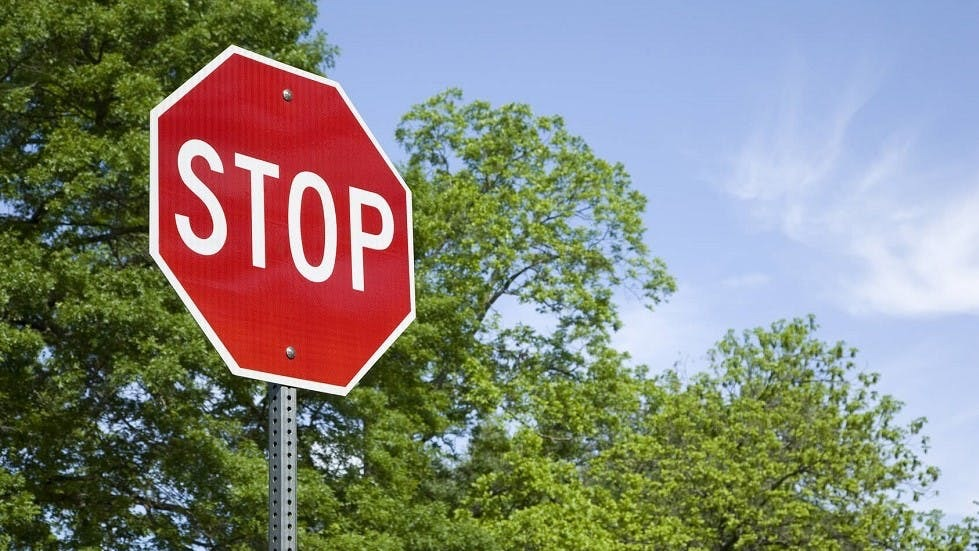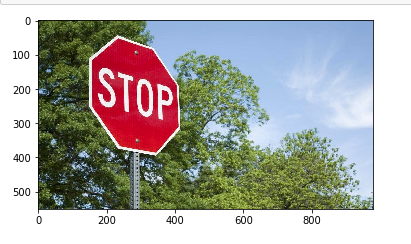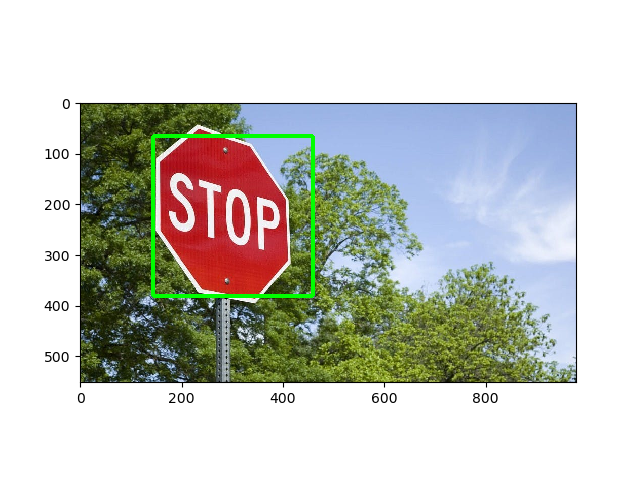# Detect an object with OpenCV-Python

OpenCV is the huge open-source library for computer vision, machine learning, and image processing and now it plays a major role in real-time operation which is very important in today’s systems. By using it, one can process images and videos to identify objects, faces, or even the handwriting of a human. This article focuses on detecting objects.

#### Object Detection

Object Detection is a computer technology related to computer vision, image processing and deep learning that deals with detecting instances of objects in images and videos. We will do object detection in this article using something known as haar cascades.

Haar Cascade classifiers are an effective way for object detection. This method was proposed by Paul Viola and Michael Jones in their paper Rapid Object Detection using a Boosted Cascade of Simple Features. Haar Cascade is a machine learning-based approach where a lot of positive and negative images are used to train the classifier.

• Positive images – These images contain the images which we want our classifier to identify.
• Negative Images – Images of everything else, which do not contain the object we want to detect.
Requirements.

• Run The following command in the terminal to install opencv.
```pip install opencv-python
```
• Run the following command to in the terminal install the matplotlib.
```pip install matplotlib
```

Note: Put the XML file and the PNG image in the same folder as your Python script.

#### Implementation

Image used:Opening an image

 `import` `cv2 ` `from` `matplotlib ``import` `pyplot as plt ` ` `  ` `  `# Opening image ` `img ``=` `cv2.imread(``"image.jpg"``) ` ` `  `# OpenCV opens images as BRG  ` `# but we want it as RGB and  ` `# we also need a grayscale  ` `# version ` `img_gray ``=` `cv2.cvtColor(img, cv2.COLOR_BGR2GRAY) ` `img_rgb ``=` `cv2.cvtColor(img, cv2.COLOR_BGR2RGB) ` ` `  `# Creates the environment  ` `# of the picture and shows it ` `plt.subplot(``1``, ``1``, ``1``) ` `plt.imshow(img_rgb) ` `plt.show() `

Output:Recognition

We will use the `detectMultiScale()` function of OpenCV to recognize big signs as well as small ones:

 `# Use minSize because for not  ` `# bothering with extra-small  ` `# dots that would look like STOP signs ` `found ``=` `stop_data.detectMultiScale(img_gray,  ` `                                   ``minSize ``=``(``20``, ``20``)) ` ` `  `# Don't do anything if there's  ` `# no sign ` `amount_found ``=` `len``(found) ` ` `  ` `  `if` `amount_found !``=` `0``: ` `     `  `    ``# There may be more than one ` `    ``# sign in the image ` `    ``for` `(x, y, width, height) ``in` `found: ` `         `  `        ``# We draw a green rectangle around ` `        ``# every recognized sign ` `        ``cv2.rectangle(img_rgb, (x, y),  ` `                      ``(x ``+` `height, y ``+` `width),  ` `                      ``(``0``, ``255``, ``0``), ``5``) `

Here is the full script for lazy devs:

 `import` `cv2 ` `from` `matplotlib ``import` `pyplot as plt ` ` `  `# Opening image ` `img ``=` `cv2.imread(``"image.jpg"``) ` ` `  `# OpenCV opens images as BRG  ` `# but we want it as RGB We'll  ` `# also need a grayscale version ` `img_gray ``=` `cv2.cvtColor(img, cv2.COLOR_BGR2GRAY) ` `img_rgb ``=` `cv2.cvtColor(img, cv2.COLOR_BGR2RGB) ` ` `  ` `  `# Use minSize because for not  ` `# bothering with extra-small  ` `# dots that would look like STOP signs ` `found ``=` `stop_data.detectMultiScale(img_gray,  ` `                                   ``minSize ``=``(``20``, ``20``)) ` ` `  `# Don't do anything if there's  ` `# no sign ` `amount_found ``=` `len``(found) ` ` `  `if` `amount_found !``=` `0``: ` `     `  `    ``# There may be more than one ` `    ``# sign in the image ` `    ``for` `(x, y, width, height) ``in` `found: ` `         `  `        ``# We draw a green rectangle around ` `        ``# every recognized sign ` `        ``cv2.rectangle(img_rgb, (x, y),  ` `                      ``(x ``+` `height, y ``+` `width),  ` `                      ``(``0``, ``255``, ``0``), ``5``) ` `         `  `# Creates the environment of  ` `# the picture and shows it ` `plt.subplot(``1``, ``1``, ``1``) ` `plt.imshow(img_rgb) ` `plt.show() `

Output :My Personal Notes arrow_drop_upCheck out this Author's contributed articles.

If you like GeeksforGeeks and would like to contribute, you can also write an article using contribute.geeksforgeeks.org or mail your article to contribute@geeksforgeeks.org. See your article appearing on the GeeksforGeeks main page and help other Geeks.

Please Improve this article if you find anything incorrect by clicking on the "Improve Article" button below.

Article Tags :
Practice Tags :

Be the First to upvote.

Please write to us at contribute@geeksforgeeks.org to report any issue with the above content.